# Computing Stirling numbers with limited integers

A couple days ago I wrote a post about a probability problem that involved calculating Stirling numbers. There are two kinds of Stirling numbers, creatively called “Stirling numbers of the first kind” and “Stirling numbers of the second kind.” The second kind come up more often in application, and so when authors say “Stirling numbers” without qualification, they mean the second kind. That’s the convention I’ll use here.

The Stirling number S(n, k) can be computed via the following series, though we will take a different approach for reasons explained below.## Problem statement

This made me think of the following problem. Suppose you want to calculate Stirling numbers. You want to use integer arithmetic in order to get exact results.

And suppose you can use integers as large as you like, but you have to specify the maximum integer size before you start calculating. Imagine you have to pay \$N to work with N-digit integers, so you want to not use a larger value of N than necessary.

## Intermediate results

There are a couple problems with using the equation above. Direct implementation of the equation would first calculate k! S(n, k) first, then divide by k! to get S(n, k). This would be costly because it would require calculating a number k! times bigger than the desired final result. (You could refactor the equation so that there is no division by k! at the end, but this gives an expression that includes non-integer terms.)

In the earlier post, I actually needed to calculate k! S(n, k) rather than S(n, k) and so this wasn’t a problem. This brings up the second problem with the equation above. It’s an alternating series, and it’s not clear whether evaluating the series produces intermediate results that are larger than the final result.

## Recursion

Stirling numbers can be computed recursively usingwith the base cases S(n, n) = 1 for n ≥ 0 and S(n, 0) = S(0, n) for n > 0. This computes Stirling numbers via a sum of smaller Stirling numbers, and so the intermediate results are no larger than the final result.

So now we’re left with the question of how big Stirling numbers can be. Before computing S(n, k) you need an upper bound on how many digits it will have.

## Bounding Stirling numbers

Stirling numbers can be bound in terms of binomial coefficients.This reduces the problem to finding an upper bound on binomial coefficients. I wrote about a simple bound on binomial coefficients here.

# Data file character frequencies

I have a little script that will print the frequency of the most common characters in a file and the number of lines. All numbers are displayed along with their factorizations. It also prints the number of non-ASCII characters.

## CSV files

These simple statistics are surprisingly useful. For example, when I ran it on an CSV file that I downloaded recently I got the following.

    ,   397907424  =  2^5 3^3 19 24239
0   58200944  =  2^4 1699 2141
2   52955465  =  5 467 22679
1   46413310  =  2 5 23 201797
3   34811225  =  5^2 1392449

Num lines:  1745208  =  2^3 3^2 24239

All ASCII characters


This strongly implies that the CSV file really is a CSV (comma-separated value) file. Sometimes you’ll get a file with a .csv extension but the separator is a tab, a pipe, or some other character.

The number of commas is a multiple of the number of lines. That’s a good sign. Apparently this is a CSV file with 12×19 columns and 1,745,208 rows . If the number of separators is not a multiple of the number of lines, maybe some lines are incomplete. Or maybe your file separator appears inside a quoted string. This is not necessarily a problem, but it means the most naive parsing won’t work.

In the file above, the most common characters, other than commas, are digits, so the file probably contains mostly numeric data.

If your file contains quotation marks, better hope it contains an even number. Even better, an even multiple of the number of lines. If not, you have some troubleshooting to do.

Incidentally, whenever the subject of CSV files comes up, someone will say “Why are you using CSV files?! Don’t you know there are better formats?” My reply is that I’m a consultant and I take data in whatever format I can get it, and that most often means a delimiter-separated text file. That works fine, except, of course, when it doesn’t.

## Unicode characters

A file with lots of non-ASCII characters is not a problem. A file with one non-ASCII character very often is a problem.

A single non-ASCII character could be an invisible character that will gum up parsing. This can be maddening to find if you’re relying on visual inspection. But if you know there’s a non-ASCII character where it shouldn’t be, such as in a file of digits and commas, then you can simply delete it.

## JSON

If you’re inspecting a JSON file, you’d expect to see lots of braces. Hopefully you have an equal number of open and close braces. But if not, you know where to being troubleshooting. You should also expect a lot of colons. Knowing the number of braces and colons gives you a clue to the structure of the file.

## Troubleshooting and guessing

When a file has no complications, the stats above tell you things you’d know from looking at the first line or two of the file. However, when there are complications, the stats can be useful.

The stats could also be useful in a context where it’s OK to make guesses. For example, you might have a script that guesses the structure of a file and proceeds accordingly. That’s fine when wrong guesses lead to obviously wrong output. It’s hard to imagine, for example, that mistaking an XML file for a CVS file would produce a subtle error.

## Related posts

 The number of fields is one more than the number of separators. Or maybe not: there may be a trailing separator after the last field. So in this case there may be 228 or 229 columns.

# Reviewing a thousand things

Suppose you’ve learned a thousand of something, maybe a thousand kanji or a thousand chemicals or a thousand species of beetles. Now you want to review them to retain what you’ve learned.

Now suppose you have a program to quiz you, drawing items from your list at random with replacement. Say you draw 100 items a day. How long until you’ve seen everything at least once?

A naive estimate would be 10 days, but that assumes you never draw the same item twice in 1000 draws. According to the birthday problem principle, there’s a 50-50 chance you’ll see a duplicate by the time you’ve made √1000 ≈ 32 draws. Drawing 100 cards a day, you’ll likely see duplicates the first day.

## Coupon collector problem

Random sampling with replacement is not an efficient way to exhaustively sample a large population. The coupon collector problem says that for a set of N items, the expected number of draws before you’ve seen everything once is approximately

N(log N + γ)

for large N. Here γ is Euler’s constant. When N = 1000 this works out to 7485. So if you draw 100 items a day, you’d expect to have seen everything after 75 days. Of course it might take more or less time than that, but that’s the average.

To put it another way, random sampling with replacement is about 7.5 times less efficient than systematic sampling if you’re concerned with expected time to exhaustive sampling.

In general, the relative inefficiency is log N + γ. So for a set of N = 100 things, for example, the relative inefficiency is about 5.2.

## Occupancy problem

We can arrive at approximately the same result using methods from a couple other posts. Last week I wrote about the occupancy problem distribution. That post defined X(N, r) and gives its mean and variance.

When we draw r random samples with replacement from a set of N items, let X(N, r) be the random variable that represents the number of distinct items in the sample. …

The mean of X(N, r) isSo if we have set of N = 1000 things and sample with replacement 7000 times, we expect to see 999 distinct items on average. Of course this is an average, so we might see less. And we might see more, but not much more!

## Probabilities

The approaches above have worked with expected values, not individual probabilities. The coupon collector problem looks at the expected number of draws needed to before you see everything. The occupancy problem looks at the expected number of things you’ve seen after a certain number of draws.

We could calculate the probability of having seen everything after r draws using the expression here.

After 7,000 draws, there’s a 40% chance we’ve seen everything. After 7,274 draws the chances of having seen everything are even, i.e. the median number of draws is 7,274, not that different from the mean number of draws estimated above.

After 10,000 draws there is a 96% chance we’ve seen everything.

## A note on computation

The expression used in the previous section relies on Stirling numbers, and these are a bit difficult to compute. The calculation involves very large integers, but we cannot use logarithms because we are adding large numbers, not multiplying them.

This post includes Python code for calculating Stirling numbers using Python’s large integer feature. But the probability we’re after is the ratio of two integers too large to convert to floating point numbers. The following code gets around this problem using the Decimal class.

    from math import comb, log10
from decimal import Decimal, getcontext

def k_factorial_stirling(n, k):
return sum((-1)**i * comb(k, i)*(k-i)**n for i in range(k+1))

def prob(N, r):
getcontext().prec = int(r*log10(N)) + 100
return Decimal( k_factorial_stirling(r, N) ) / Decimal( N**r)

print( round(prob(1000,  7000), 4) )
print( round(prob(1000,  7274), 4) )
print( round(prob(1000, 10000), 4) )



How do we know how many decimal places we’ll need? We’re computing a probability, so the denominator is larger than the numerator. We estimate the number of digits in the denominator, then throw in 100 more just for good measure.

# Comparing approximations for ellipse perimeter

This post will compare the accuracy of approximations for the perimeter of an ellipse.

The exact perimeter is given in terms of an elliptic integral. (That’s where the elliptic integrals  gets their name.) And so an obvious way to approximate the perimeter would be to expand the elliptic integral in a power series. Unfortunately this series converges slowly for highly eccentric ellipses.

Ernst Kummer found a series that converges more quickly . Let a and b be the semi-major and semi-minor axes of an ellipse and letThen Kummer’s series isThe following plot compares the accuracy of truncating Kummer’s series after powers of x equal to 1 through 4.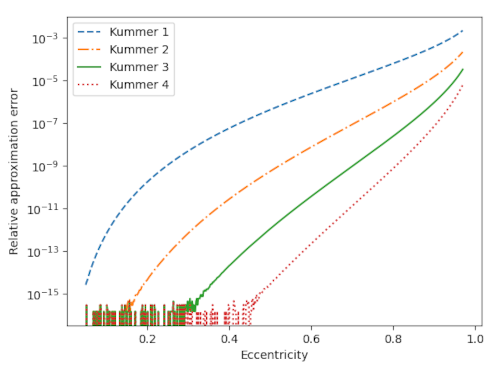The noise at the bottom of the chart comes from the limits of machine precision. For moderate values of eccentricity, three terms of Kummer’s series gives an approximation so accurate that all the error is coming from the limitations of floating point resolution.

I’ve written about approximations for the perimeter of an ellipse before. The approximation based on the 3/2 norm is about as accurate as Kummer’s series truncated after the x term. The error in Ramanujan’s approximation is between that of Kummer’s series with the cubic and quartic terms.

For the orbit of Pluto (e = 0.2488) the first-order Kummer approximation is good to 9 figures and the higher-order approximations are essentially good to machine precision.

For the orbit of Haley’s comet (e = 0.9671) the successive Kummer approximations are good to 2, 3, 4, and 5 figures.

 Carl E. Linderholm and Arthur C. Segal. An Overlooked Series for the Elliptic Perimeter. Mathematics Magazine, Vol. 68, No. 3 (Jun., 1995), pp. 216–220.

# Instant classic

“Instant classic” is, of course, an oxymoron. A classic is something that has passed the test of time, and by definition that cannot happen instantly.

But how long should the test of time last? In his book Love What Lasts, Joshua Gibbs argues that 100 years after the death of the artist is about the right amount of time, because that is the span of personal memory.

An individual may have memories from 50 years ago that he passes on to someone 50 years younger, but it’s unlikely the young hearer will pass along the same memory. Or to look at it another way, 100 years is about four generations, and hardly anyone has much connection to a great great grandparent.

If a work is still of interest 100 years after the death of the person who created it, the work must have some value that extends beyond a personal connection to its creator.

I’m about a third of the way through Gibbs’ book and it’s the most thought-provoking thing I’ve read lately.

# Occupancy problem distribution

Suppose you have a random number generator that returns numbers between 1 and N. The birthday problem asks how many random numbers would you have to output before there’s a 50-50 chance that you’ll repeat a number. The coupon collector problem asks how many numbers you expect to generate before you’ve seen all N numbers at least once.

These are examples of occupancy problems. The name comes from imagining N urns, randomly assigning balls to each, then asking how many urns are occupied.

Suppose people are walking into a room one at a time. The birthday problem asks at what point is there even odds that two people in the room have the same birthday. The coupon collector problem asks the expected number of people to enter the room before all birthdays are represented. But we could ask, for example, after 100 people enter the room, how likely it is that there are 70 different birthdays represented.

When we draw r random samples with replacement from a set of N items, let X(N, r) be the random variable that represents the number of distinct items in the sample. For example, suppose a hashing algorithm returns one of N possible hash codes. The number of distinct hash codes after hashing r documents would be X(N, r).

The probability mass function (pmf) for X(N, r) has been calculated, for example in , but it’s very complicated and you’re not likely to get much understanding of X(N, r) by looking at the expression for the pmf. The mean and variance of X(N, r) are somewhat complicated , but easier to work with than the pmf.

The mean of X(N, r) isIn the special case that N = r and N is large, the mean is approximately N(1 – 1/e). For example, suppose you had a deck of 52 cards. You draw one card, put it back in the deck, and shuffle the deck. Repeat this 52 times. You would get about 33 distinct cards on average.

The variance of X(N, r) is more complicated than the mean.As with the mean, the case N = r with N large is simpler. In that case the variance is approximately N(1/e – 1/e²). In the example above, this works out to about 12. The standard deviation is about 3.5, and so you’d often see 33 ± 7 distinct cards.

## Related posts

 Paul G. Hoel, Sidney C. Port, and Charles J. Stone, Introduction to Probability TheoryX(N, r), Houghton Mifflin, Boston, 1971, pp. 43–45.

 Emily S. Murphree. Replacement Costs: The Inefficiencies of Sampling with Replacement. Mathematics Magazine, Vol. 78, No. 1 (Feb., 2005), pp. 51-57.

# Hypergeometric distribution symmetry

One of these days I’d like to read Feller’s probability book slowly. He often says clever things in passing that are easy to miss.

Here’s an example from Feller  that I overlooked until I saw it cited elsewhere.

Suppose an urn contains n marbles, n1 red and n2 black. When r marbles are drawn from the urn, the probability that precisely k of the marbles are red follows a hypergeometric distribution.Feller mentions that this expression can be rewritten asWhat just happened? The roles of the total number of red marbles n1 and the sample size r, have been swapped.

The proof is trivial—expand both expressions using factorials and observe that they’re equal—but that alone doesn’t give much insight. It just shows that two jumbles of symbols represent the same quantity.

The combinatorial interpretation is more interesting and more useful. It says that you have two ways to evaluate the probability: focusing on the sample or focusing on the population of red marbles.

The former perspective says we could multiply the number of ways to choose k marbles from the supply of n1 red marbles and rk black marbles from the supply of nn1 black marbles, then divide by the number of ways to choose a sample of r marbles from an urn of n marbles.

The latter perspective says that rather than partitioning our sample of r marbles, we could think of partitioning the population of red marbles into two groups: those included in our sample and those not in our sample.

I played around with adding color to make it easier to see how the terms move between the two expressions. I colored the number of red marbles red and the sample size blue.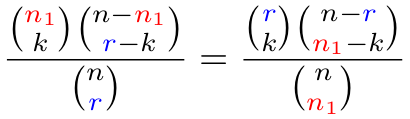Moving from left to right, the red variable goes down and the blue variable goes up.

## Related posts

 William Feller. An Introduction to Probability Theory and Its Applications. Volume 1. Third edition. Page 44.

# AM over GM

Suppose you take the arithmetic mean and the geometric mean of the first n integers. The ratio of these two means converges to e/2 as n grows . In symbols,Now suppose we wanted to visualize the convergence by plotting the expression on the left side for a sequence of ns.

First let’s let n run from 1 to 100.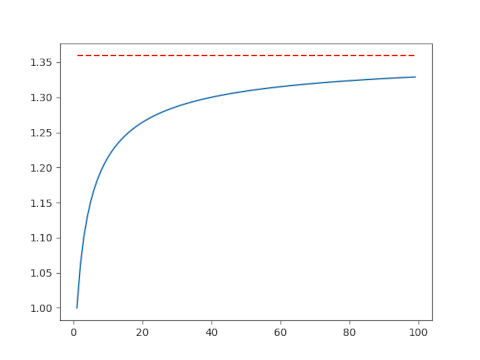This isn’t very convincing. Maybe the convergence is just slow, or maybe it’s not actually converging to e/2. Let’s make another plot including larger values of n. This will require a little additional work.

## Avoiding overflow

Here’s the code that made the plot above.

    import matplotlib.pyplot as plt
import numpy as np
from scipy.special import factorial

AM = lambda n: (n+1)/2
GM = lambda n: factorial(n)**(1/n)
n = np.arange(1, 100)
plt.plot(n, AM(n)/GM(n))
plt.plot(n, 0*n + np.exp(1)/2, 'r--')


This works for n up to 170, but it fails when n = 171 because at that point factorial overflows.

This is a very common situation. We ultimately want to compute a fairly small number, but we encounter extremely large numbers in an intermediate step.

We need to avoid directly calculating n! before taking the nth root. The way to do this is to work with logarithms. Now n! = Γ(n+1) and SciPy has a function for computing the log of the gamma function directly without first computing the gamma function, thus avoiding overflow.

We replace GM above with GM2 below:

    GM2 = lambda n: np.exp(gammaln(n+1)/n)

Now we compute the geometric mean of the first n natural numbers for very large values of n. We only need n = 1000 to see convergence, but the code could handle much larger values of n without overflowing.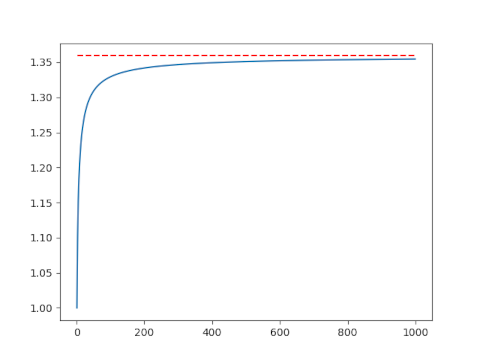## Related posts

 Richard P. Kubelka. Means to an End. Mathematics Magazine, Vol. 74, No. 2 (April 2001), pp. 141–142

# Category theory without categories

I was bewildered by my first exposure to category theory. My first semester in graduate school I had a textbook with definitions like “A gadget is an object G such that whenever you have this unfamiliar constellation of dots and arrows, you’re allowed to draw another arrow from here to there.” What? Why?!

I revisited category theory occasionally after college, going through cycles of curiosity followed by revulsion. It took several cycles before I could put my finger on why I found category theory so foreign.

There are numerous obstacles to appreciating category theory, but the biggest may be diagrammatic reasoning. More specifically, having to learn diagrammatic reasoning at the same time as facing other challenges.

Why should diagrammatic reasoning be difficult? Isn’t the purpose of diagrams to make things clearer?

Usually diagrams are supplementary. They illustrate things that are described verbally. But in category theory, the diagrams are primary, not supplementary. Instead, you have definitions and theorems stated in the language of diagrams .

In practice, category theory uses a style of presentation you’re unlikely to have seen anywhere else. But this is not essential. You could do category theory without drawing diagrams, though nobody does. And, importantly for this post, you can use category theory-like diagrams without reference to categories. That’s what William Lawvere does in his book Conceptual Mathematics. The book uses Karate Kid-like pedagogy: the student gains fluency with a practice before being told its significance.

When you see a triangle made of two arrows, what’s the significance of the existence of an arrow that makes the diagram into a triangle? What difference does it make when the missing arrow goes on one side of the diagram or the other as in the two diagrams below?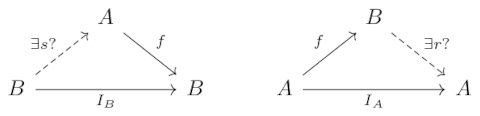The answer is not obvious if you’re unfamiliar with this way of thinking, and yet the problem has nothing to do with category theory per se. You could ask the question in the context of finite sets and functions. And that’s what Lawvere’s book does, acquainting the reader with diagrammatic reasoning before getting into category theory as such.

***

 Or more to the heart of the matter, you have definitions in terms of functions (“morphisms”) that are represented by diagrams. The difficulty does not come from the diagrams but rather from formulating things in terms of the existence and uniqueness of functions rather than more tangible arguments about sets and elements. This indirect formulation may seem unnecessary, or even sadistic, but it is generalizes further.

# Contraharmonic mean

I’ve mentioned the harmonic mean multiple times here, most recently last week. The harmonic mean pops up in many contexts.

The contraharmonic mean is a variation on the harmonic mean that comes up occasionally, though not as often as its better known sibling.

## Definition

The contraharmonic mean of two positive numbers a and b isand more generally, the contraharmonic mean of a sequence of positive numbers is the sum of their squares over their sum.

## Why the name?

What is “contra” about the contraharmonic mean? The harmonic mean and contraharmonic mean have a sort of reciprocal relationship. The ratioequals a/b when m is the harmonic mean and it equals b/a when m is the contraharmonic mean.

## Geometric visualization

Construct a trapezoid with two vertical sides, one of length a and another of length b. The vertical slice through the intersection of the diagonals, the dashed blue line in the diagram below, has length equal to the harmonic mean of a and b.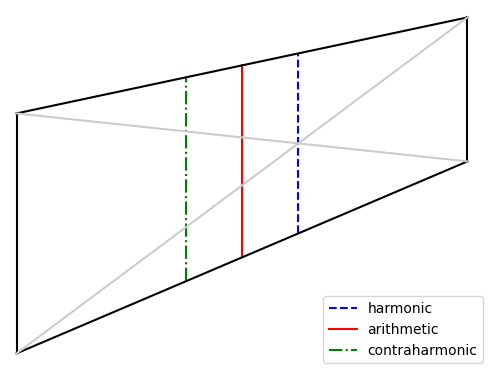The vertical slice midway between the two vertical sides, the solid red line in the diagram, has length equal to the arithmetic mean of a and b.

The vertical slice on the opposite side of the red line, as from the red line on one side as the blue line is on the other, the dash-dot green line above, has length equal to the contraharmonic mean of a and b.

## Connection to statistics

The contraharmonic mean of a list of numbers is the ratio of the sum of the squares to the sum. If we divide the numerator and denominator by the number of terms n we see this is the ratio of the second moment to the first moment, i.e. the mean of the squared values divided the mean of the values.

This smells like the ratio of variance to mean, known as the index of dispersion D, except variance is the second central moment rather than the second moment. The second moment equals variance plus the square of the mean, and soand